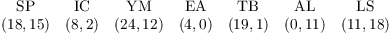# Substitution Ciphers and Block Ciphers

• A cipher takes a plaintext and encodes it to produce a ciphertext.
• In many cryptographic systems, the letters of the alphabet are represented by the numbers 0, 1, ..., 25.
• In a substitution cipher, characters in the plaintext are replaced by other characters.
• Affine transformations can be used to produce simple substitution ciphers.
• In a block cipher, blocks of characters are replaced by blocks of characters.
• Affine matrix transformations can be used to produce simple block ciphers.

A cipher takes a message (the plaintext) and encodes it --- puts it in a form (the ciphertext) where the information in the message is not obvious upon inspection. The recipient of the message takes the ciphertext and decodes it --- performs an operation which recovers the plaintext from the ciphertext.

Example. (A shift cipher) This is also known as a Caesar cipher, since it was supposedly used by Julius Caesar.

The letters of the alphabet will be represented by the numbers 0, ..., 25:I won't make a distinction between upper and lower case. If x is a letter, I'll encode it using(I could use any nonzero number from 1 to 25 in place of 11.) The formula above replaces each letter with another letter; in effect, the alphabet gets "shifted" 11 places to the left.

The translation table for this cipher is:For example, consider the message"I" is replaced by "T", "W" by "H", and so on. I get the ciphertextI'll group the letters into 5-letter words to hide the original word groupings:I've thrown in an extra "Z" at the end to make the last group come out evenly. If you decode the message, you'd getYou can see that the 5-letters grouping and the extra "Z" did no harm, since it's evident what the plaintext was.

Note that the equationcan be solved for x:This equation is the decoding transformation; it's equivalent to reading the translation table backward.Example. If you use the shiftthe messageI wrote a computer program to do this. There are only 26 possible shifts, so if you wanted to decode this by brute force, you could feed the ciphertext through 26 shift programs and see which one produced a sensible message. Shift ciphers are not of much use when it comes to protecting secrets!The next thing to try is an affine transformation:I need the last condition in order to ensure that I can decode messages. This is equivalent to being able to invert the transformation. Now if, then a is invertible mod 26, soThis equation can be used to decode messages.

Example. Consider the transformation. To find the decoding transformation, solve for x in terms of y:Here's the translation table:The plaintextgives the ciphertextGroup the letters:Shift ciphers and affine transformation ciphers are called substitution or character ciphers because each letter is replaced by another letter. They're simple to use, but relatively easy to crack. For example, with any reasonably large message you can count the letters in the ciphertext and guess the substitution using frequency tables for letters in the English language.

As a partial remedy to frequency analysis, you might think of enciphering blocks of k letters at a time. To do this, encode letters as number from 0 to 25 in the usual way. Consider a block of k letters. As the cipher key, choose amatrix M which is invertible mod 26. (M will be invertible mod 26 ifis relatively prime to 26.) Then the cipher transformation is, i.e.You can decipher messages using.

Example. (a digraphic cipher) TakeSinceand, M is invertible mod 26. In fact,(Note that, because.)

Here's a message:Break it up into two-letter groups and convert them to 2-dimensional vectors:Finally, use M to encode each vector. For example,, so the first two letters of the ciphertext are "FD".

Continuing in this way, you obtain the ciphertextContact information

Copyright 2007 by Bruce Ikenaga